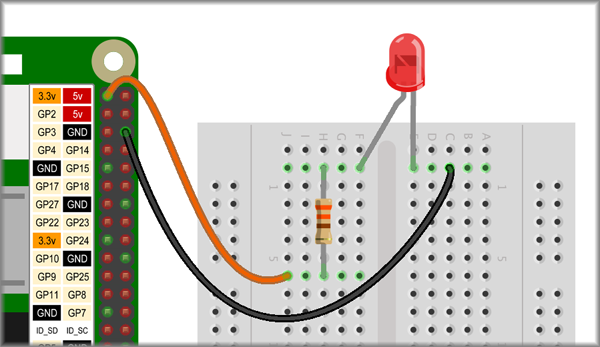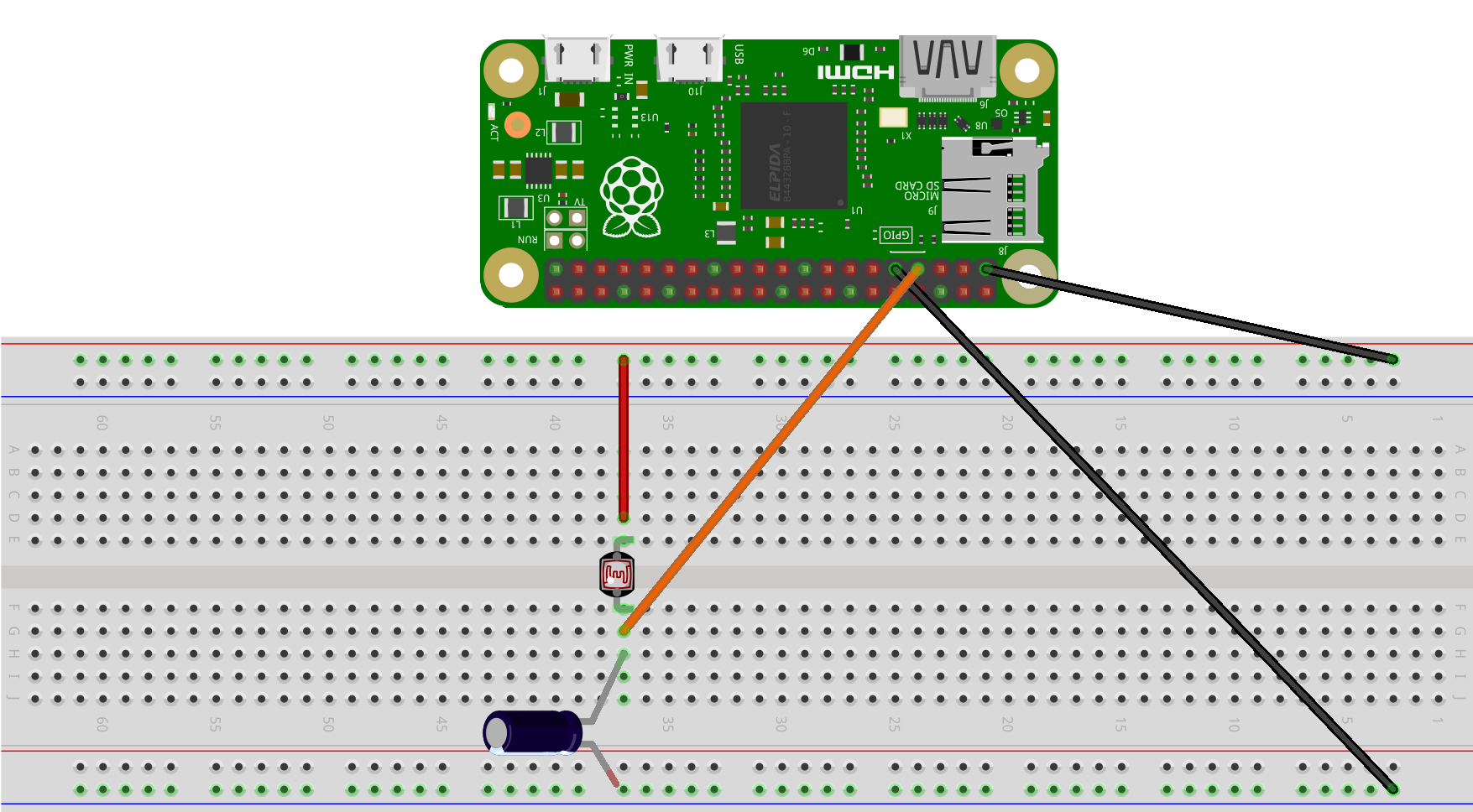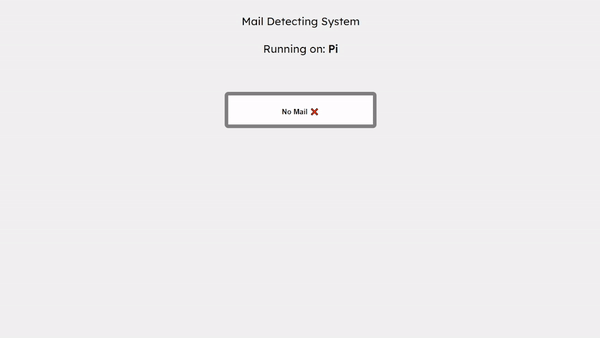So a couple of weeks ago I saw myself checking the mailbox frequently and I thought to myself: “Is there a more lazy easier way to do this?”.

A couple of weeks ago I made a project called Spying on the Sun using a Photoresistor and I thought that I could use the same principle to detect if there is mail in the mailbox.

Think about it for a second.

We can constantly shine a LED on the photoresistor if there is mail in the mailbox, the photoresistor will be covered by the mail and the voltage will drop. If there is no mail, the photoresistor will be exposed to the LED and the voltage will rise.

So I am gonna use the same Raspberry PI Zero W and the same photoresistor that I used in the previous project.

So first we will need to also connect a LED to the Raspberry PI Zero W.

I Followed this amazing guide by Raspberry Pi Projects to connect the LED to the Raspberry PI Zero W.This uses 2 wires for positive and negative and a 330-ohm resistor connected to the anode (Longer leg) side of the LED.

We can also use the same connections from the last project for the photoresistor.For more information about the wiring and how the photoresistor works, check out the previous project Spying on the Sun using a Photoresistor.

So now we have the LED and the photoresistor connected to the Raspberry PI Zero W.

We can now write the code to detect if there is mail in the mailbox we will take the code from the previous project and modify it.

``````from flask import Flask
import RPi.GPIO as GPIO
import time

GPIO.setmode(GPIO.BOARD)

resistorPin = 7

light_values = []
light_times = []

@app.route('/')
def index():
html = """
<script src="https://cdn.jsdelivr.net/npm/chart.js"></script>
<canvas id="myChart" width="400" height="400"></canvas>
<script>
const labels = """ + str(light_times) + """;
const ctx = document.getElementById('myChart');

new Chart(ctx, {
type: 'line',
data: {
labels: labels,
datasets: [{
label: 'Light Values',
data: """ + str(light_values) + """,
fill: false,
borderColor: 'rgb(75, 192, 192)',
tension: 0.1
}]
},
options: {
scales: {
y: {
beginAtZero: true,
reverse: true
}
}
}
});
</script>
"""
return html

while True:
GPIO.setup(resistorPin, GPIO.OUT)
GPIO.output(resistorPin, GPIO.LOW)
time.sleep(0.1)

GPIO.setup(resistorPin, GPIO.IN)
currentTime = time.time()
diff = 0

while(GPIO.input(resistorPin) == GPIO.LOW):
diff  = time.time() - currentTime

light_values.append(diff * 1000) # Add the time it took to charge the capacitor to the list
light_times.append(time.strftime("%H:%M:%S")) # Add the current time to the list
print(diff * 1000)
time.sleep(1)

if __name__ == '__main__':
app.run()
``````

We will not be using any graphs or threading in this project, so we can remove the code that is not needed.

``````from flask import Flask
import RPi.GPIO as GPIO
import time

GPIO.setmode(GPIO.BOARD)

resistorPin = 7

light_values = []
light_times = []

@app.route('/')
def index():
html = """
<title> Mail Detection </title>

<body>
<h1> Mail Detection </h1>
</body>
"""
return html

while True:
GPIO.setup(resistorPin, GPIO.OUT)
GPIO.output(resistorPin, GPIO.LOW)
time.sleep(0.1)

GPIO.setup(resistorPin, GPIO.IN)
currentTime = time.time()
diff = 0

while(GPIO.input(resistorPin) == GPIO.LOW):
diff  = time.time() - currentTime

light_values.append(diff * 1000) # Add the time it took to charge the capacitor to the list
light_times.append(time.strftime("%H:%M:%S")) # Add the current time to the list
print(diff * 1000)
time.sleep(1)

if __name__ == '__main__':
app.run(host="", debug=True)
``````

Now we will also not continuously check if there is mail in the mailbox, we will only check if there is mail when we visit the website. We can do that by calling the function read_photoresistor and returning the value of the photoresistor when asked.

``````from flask import Flask
import RPi.GPIO as GPIO
import time

GPIO.setmode(GPIO.BOARD)

resistorPin = 7

light_values = []
light_times = []

@app.route('/')
def index():
html = """
<title> Mail Detection </title>

<body>
<h1> Mail Detection </h1>
</body>
"""
return html

time.sleep(1)
GPIO.setup(resistorPin, GPIO.OUT)
GPIO.output(resistorPin, GPIO.LOW)
time.sleep(0.1)

GPIO.setup(resistorPin, GPIO.IN)
currentTime = time.time()
diff = 0

while(GPIO.input(resistorPin) == GPIO.LOW):
diff  = time.time() - currentTime

light_values.append(diff * 1000) # Add the time it took to charge the capacitor to the list
light_times.append(time.strftime("%H:%M:%S")) # Add the current time to the list
return (diff * 1000)

if __name__ == '__main__':
app.run(host="", debug=True)
``````

We will also not be storing any values or times as they are not needed.

``````from flask import Flask
import RPi.GPIO as GPIO
import time

GPIO.setmode(GPIO.BOARD)

resistorPin = 7

@app.route('/')
def index():
html = """
<title> Mail Detection </title>

<body>
<h1> Mail Detection </h1>
</body>
"""
return html

time.sleep(1)
GPIO.setup(resistorPin, GPIO.OUT)
GPIO.output(resistorPin, GPIO.LOW)
time.sleep(0.1)

GPIO.setup(resistorPin, GPIO.IN)
currentTime = time.time()
diff = 0

while(GPIO.input(resistorPin) == GPIO.LOW):
diff  = time.time() - currentTime

light_values.append(diff * 1000) # Add the time it took to charge the capacitor to the list
light_times.append(time.strftime("%H:%M:%S")) # Add the current time to the list
return (diff * 1000)

if __name__ == '__main__':
app.run(host="", debug=True)
``````

Now another trick I want to show before we do the code to check if there is mail is that if we want our website to be able to be seen by anything other than our computer we need to add the host IP to the `host=""` in `app.run`.

Instead of manually finding the ip and adding it to the code we can use the following code to find the IP of the raspberry pi.

``````import socket

hostname=socket.gethostname()
s = socket.socket(socket.AF_INET, socket.SOCK_DGRAM)
s.connect(("8.8.8.8", 80))
``````

This code will find the ip of the raspberry pi and store it in the variable IPAddr. It will also store the hostname of the raspberry pi in the variable hostname.

Now we can use this code to check if there is mail in the mailbox.

``````from flask import Flask
import RPi.GPIO as GPIO
import time
import socket

hostname=socket.gethostname()
s = socket.socket(socket.AF_INET, socket.SOCK_DGRAM)
s.connect(("8.8.8.8", 80))

GPIO.setmode(GPIO.BOARD)

resistorPin = 7

@app.route('/')

def index():
html = """
<title>Mail Detect</title>
<style>
body {
font-family: Arial, Helvetica, sans-serif;
background-color: #f1f1f1;
}
.box {
width: 400px;
height: 50px;
background-color: #fff;
border: 1px solid #000;
margin: 0 auto;
margin-top: 100px;
text-align: center;
font-size: 20px;
font-weight: bold;
}
.title {
font-size: 30px;
font-family: 'Lexend';
}
</style>

<p>
&nbsp;
</p>
<center>
<div class="title">Mail Detecting System <br><br> Running on: <b>"""+ hostname + """</b></div>
"""
if light > 100: # We check if the light value is greater than 100 which means something is blocking the light.
html += '<div class="box" style="border: 10px solid #98e5e5">Mail Detected ✉️'
else:
html += '<div class="box" style="border: 10px solid gray"> No Mail ❌'

html += """

<pre> """ + str(light) + """ </pre>

</div>
</center>
"""
return html

time.sleep(1)
GPIO.setup(resistorPin, GPIO.OUT) # Set the resistorPin to output mode so we can charge the capacitor
GPIO.output(resistorPin, GPIO.LOW) # Set the resistorPin to low so we can charge the capacitor
time.sleep(0.1)

GPIO.setup(resistorPin, GPIO.IN) # Set the resistorPin to input mode so we can read the voltage
currentTime = time.time() # Get the current time
diff = 0

while(GPIO.input(resistorPin) == GPIO.LOW): # While the voltage is low keep looping until the voltage is high
diff  = time.time() - currentTime # Get the difference between the current time and the time when the voltage was low

return (diff * 1000) # Print the time it took to charge the capacitor in milliseconds(ms)

if __name__ == '__main__':
``````

Now we can go to the ip of the raspberry pi and see if there is mail in the mailbox.Now there is a problem with this code. It will not update if there is a mail or not unless you refresh the page.

To fix this we can make a sort of API using the `app.route()` where it will have the value of the photoresistor so we can continuously call that API without needing to refresh the page.

``````from flask import Flask
import RPi.GPIO as GPIO
import time
import socket

hostname=socket.gethostname()
s = socket.socket(socket.AF_INET, socket.SOCK_DGRAM)
s.connect(("8.8.8.8", 80))

GPIO.setmode(GPIO.BOARD)

resistorPin = 7

@app.route('/')

def index():
html = """
<title>Mail Detect</title>
<style>
body {
font-family: Arial, Helvetica, sans-serif;
background-color: #f1f1f1;
}
.box {
width: 400px;
height: 50px;
background-color: #fff;
border: 1px solid #000;
margin: 0 auto;
margin-top: 100px;
text-align: center;
font-size: 20px;
font-weight: bold;
}
.title {
font-size: 30px;
font-family: 'Lexend';
}
</style>

<p>
&nbsp;
</p>
<center>
<div class="title">Mail Detecting System <br><br> Running on: <b>"""+ hostname + """</b></div>
"""
if light > 100:
html += '<div class="box" style="border: 10px solid #98e5e5">Mail Detected ✉️'
else:
html += '<div class="box" style="border: 10px solid gray"> No Mail ❌'

html += """
</div>
</center>
<script>
setInterval(function() {
fetch('/api/v1/light')
.then(response => response.text())
.then(data => {
if (data > 100) {
document.querySelector('.box').style.border = '10px solid #98e5e5';
document.querySelector('.box').innerHTML = 'Mail Detected ✉️';
} else {
document.querySelector('.box').style.border = '10px solid gray';
document.querySelector('.box').innerHTML = 'No Mail ❌';
}
});
}, 2000);
</script>
"""
return html

@app.route('/api/v1/light') # We use the route /api/v1/light to get the value of the photoresistor
def light():

time.sleep(1)
GPIO.setup(resistorPin, GPIO.OUT)
GPIO.output(resistorPin, GPIO.LOW)
time.sleep(0.1)

GPIO.setup(resistorPin, GPIO.IN) #
currentTime = time.time()
diff = 0

while(GPIO.input(resistorPin) == GPIO.LOW):
diff  = time.time() - currentTime

return (diff * 1000)

if __name__ == '__main__':